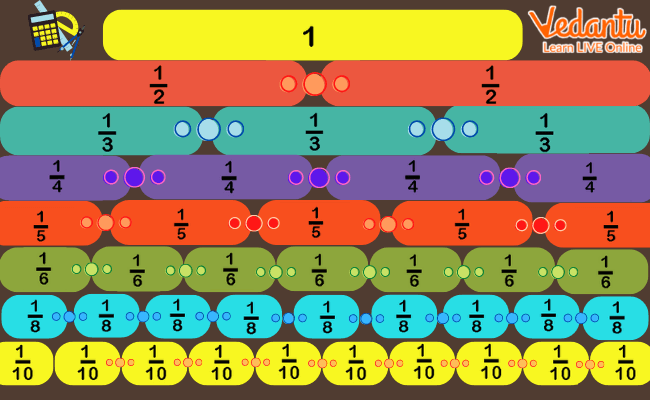Courses
Courses for Kids
Free study material
Free LIVE classes
More

# Fractions in Year 4 for Kids (Age Group of 8-9 Years)## An introduction to Fractions for Kids in Year 4

Who likes to have pizzas and apple pies? Is that you? Well, most of us enjoy having pizzas and pies, and here we will see how these little joyful things help us learn fractions. A fraction represents a part of a whole. Well, you must have noticed that a pizza is divided into several pieces, say 4, 6, 8, or 12; when you have 2 out of 4 pieces, then you’re having $\frac{1}{2}$ of the pizza. Similarly, when you’re having 3 of the 8 pieces of a pie, you are having $\frac{3}{8}$ of the whole pie.

Now let us learn more about fractions in year 4 for kids, in the following sections, which will make the concepts easy to understand. You will be able to relate to the concept of fractions and apply it while solving sums.

## What is a Fraction?

As stated above, a fraction represents a part of a whole. When something is divided into several parts, the fraction indicates how many of those parts you have.

For example, if you have 4 chocolates from a box containing 15 chocolates, the fraction of chocolates that you ate can be written as $\frac{4}{5}$ where the numerator, 4 represents how many parts you have eaten and the denominator 15 represents how many equal parts is the whole box of chocolates is divided into.

## Methods of Teaching Fractions to Kids

There are lots of methods that you can follow to teach your child and to make them understand fractions. Some of the easy methods are as follows.

### 1. Explore Equivalent Fractions

When different fractions yield the same value, they are called equivalent fractions. For example, $\frac{3}{6}$ and $\frac{1}{2}$ are equivalent fractions. The equivalent fraction can be taught to a kid using fraction walls. For example, you can ask your child to make a fraction wall to work out that $\frac{1}{3}$ is equivalent to $\frac{2}{6}$, $\frac{3}{9}$, $\frac{4}{12}$ and so on. A fraction wall is shown below where 1 is divided into fractions.Fraction Wall

### 2. Simplify Fraction

This is an important concept that children should know in year 4. Simplifying fractions means reducing a fraction into its simplest form. In order to get a simplified fraction, kids are needed to find the highest common factor of the numerator and the denominator. The next step is to divide both numbers by the highest common factor. The highest common factor of a fraction number is defined as the biggest number that can be divided equally into both the numerator as well as the denominator.

For example: If we want to simplify $\frac{8}{16}$. In this fraction number 8 is the highest common factor because it is the highest number that can be divided by 8 and 16. We can therefore divide the numerator and the denominator by 8 to get the answer: $\frac{1}{2}$.

### 3. Learning Fraction while Shopping

The best example and also a teaching method of fractions can be while you take kid for shopping. For example, ask your kids to buy oranges for them. If your kid consume an orange a day and the shopkeeper sells 6 oranges in a box, ask your kid to calculate what fraction of oranges will he have in a day. Therefore, if you buy a box of 6 oranges, the fraction of oranges your kid will have in a day is $\frac{1}{6}$.

## Conclusion

This information will definitely be useful for kids who are confused about how to learn the concept of fractions. Also, parents and teachers can take a cue from these methods. Understanding fractions and related concepts can be learned by practising fraction for year 4 worksheets as well.

Last updated date: 22nd Sep 2023
Total views: 130.8k
Views today: 2.30k

## FAQs on Fractions in Year 4 for Kids (Age Group of 8-9 Years)

1. Which concepts are required to teach a child of age group 8-9 years?

Fraction is a concept that should be introduced slowly to a kid. In the initial phase of learning, fractions should be taught with real-life examples and visualization of figures. Later when a child understands the basic idea, other related concepts should be introduced such as decimal numbers, rounding of numbers, types of fractions etc.

2. What is the need for teaching fractions for year 4 kids?

Fraction for year 4 kids is an important concept learning which will help kids solve sums of many other chapters. In our daily life, we see examples of fractions. Children are required to learn these concepts to do daily life calculations. Learning the concept of how to solve multiplication and division of fractions, will be a help when they go to higher classes and solve complex numerical problems.